## TI 83 BASIC Tutorial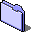# Equations and Variables

Welcome to chapter 3, now we will learn about equations and variables and how to use them. Equations are important because thay solve math problems. That is why we use calulators. (If you bought a TI83 to play games, you should have gotten a gameboy.)

### Equations

An equation is as follows: 2+2=4. This is a pretty simple. Then we have more complex equations: 2+(3*4)/2, 4+a=5, sin(25)*n=45.4, etc... We will start with simple ones. At the user screen, type 2+2. You should get 4. DUH. Now we will type in 4*3+6, and then type in4*(3+6). You should get different answers. This is due to the order of operations. For those who don't know, or don't remeber, here they are:

1. Operations in Parethesizes "( )"
2. MATH menu operations, like abs(), sqrt(), etc... (NOT >frac)
3. Multiplication and division from left to right
4. Addition and subtraction from left to right
 Example of inputing an equation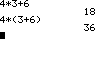### The ANS and ENTRY keys

You can recall an answer from the last equation you put in. Press: [2ND] [(-)] (ANS). This will put ANS on your screen. This variable stores the answer to the last equation you put in. If you want to recall a whole equation, press [2ND] [ENTER] (ENTRY). And the whole last equation you entered in will be on screen. If you press (ENTRY)* you will see the second to last equation you put in.
* = (From now on, any fuction that requires the [2ND] key (in yellow on the keyboard) will be in parethesizes and in bold)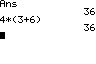### Variables

Variable are place holder for numbers. They are inmportant in that they store answers to equations and they can be used in place of numbers in equations. Here is how you store a number in a variable: 2+2->A (Press [STO>] for ->) You can use any letter to store an answer or number. That means you can store up to 26 numbers in variables. (You cannot use words as variables, like HI, the calculator interprets this as multiplication (H*I).) To recall the contents of a variable type its letter. A will produce 4 on screen. You can also use them in other equations, like: A-2 and press [ENTER] you will get 2.
 Storing an answer in a variable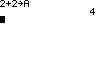Recalling the contents of a variable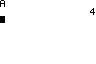Using a variable in an equation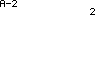### Variable Types

As I told you before, variables hold numbers. However, this is a over simplified view. On the TI 83, variables may hold complex numbers, lists, strings (text like "Hello") , etc. Don't worry about this, as they will not come up often, until chapter 9 (Advanced topics). I will expand greatly on these then.